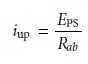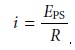Home | | Modern Analytical Chemistry | Classification of Electrochemical Methods

# Classification of Electrochemical Methods

1. Interfacial Electrochemical Methods 2. Controlling and Measuring Current and Potential

Classification of Electrochemical Methods

Although there are only three principal sources for the analytical signal—potential, current, and charge—a wide variety of experimental designs are possible;. The simplest division is be- tween bulk methods, which measure properties of the whole solution, and interfa- cial methods, in which the signal is a function of phenomena occurring at the inter- face between an electrode and the solution in contact with the electrode. The measurement of a solution’s conductivity, which is proportional to the total con- centration of dissolved ions, is one example of a bulk electrochemical method. A determination of pH using a pH electrode is one example of an interfacial electro- chemical method. Only interfacial electrochemical methods receive further consid- eration in this text.

## Interfacial Electrochemical Methods

The diversity of interfacial electrochemical methods is evident from the partial family tree shown in Figure 11.1. At the first level, interfacial electrochemical methods are divided into static methods and dynamic methods. In static methods no current passes between the electrodes, and the concentrations of species in the electrochemical cell remain unchanged, or static. Potentiometry, in which the po- tential of an electrochemical cell is measured under static conditions, is one of the most important quantitative electrochemical methods.The largest division of interfacial electrochemical methods is the group of dy- namic methods, in which current flows and concentrations change as the result of a redox reaction. Dynamic methods are further subdivided by whether we choose to control the current or the potential. In controlled-current coulometry, we completely oxidize or reduce the analyte by passing a fixed current through the analytical solution. Controlled-potential methods are subdivided further into controlled-potential coulometry and amperometry, in which a constant potential is applied during the analysis, and voltammetry, in which the potential is systematically varied.

## Controlling and Measuring Current and Potential

Electrochemical measurements are made in an electrochemical cell, consisting of two or more electrodes and associated electronics for controlling and measuring the current and potential. In this section the basic components of electrochemical in- strumentation are introduced. Specific experimental designs are considered in greater detail in the sections that follow.

The simplest electrochemical cell uses two electrodes. The potential of one of the electrodes is sensitive to the analyte’s concentration and is called the working, or indicator electrode. The second electrode, which is called the counter electrode, serves to complete the electric circuit and provides a reference potential against which the working electrode’s potential is measured. Ideally the counter electrode’s potential remains constant so that any change in the overall cell potential is attrib- uted to the working electrode. In a dynamic method, where the passage of current changes the concentration of species in the electrochemical cell, the potential of the counter electrode may change over time. This problem is eliminated by replacing the counter electrode with two electrodes: a reference electrode, through which no current flows and whose potential remains constant; and an auxiliary electrode that completes the electric circuit and through which current is allowed to flow.

Although many different electrochemical methods of analysis are possible (Fig- ure 11.1) there are only three basic experimental designs: (1) measuring the potential under static conditions of no current flow; (2) measuring the potential while con- trolling the current; and (3) measuring the current while controlling the potential. Each of these experimental designs, however, is based on Ohm’s law that a current, i, passing through an electric circuit of resistance, R, generates a potential, E; thus

E = iR

Each of these experimental designs also uses a different type of instrument. To aid in understanding how they control and measure current and potential, these in- struments are described as if they were operated manually. To do so the analyst observes a change in current or potential and manually adjusts the instrument’s set- tings to maintain the desired experimental conditions. It is important to understand that modern electrochemical instruments provide an automated, electronic means of controlling and measuring current and potential. They do so by using very differ- ent electronic circuitry than that shown here.

### Potentiometers

Measuring the potential of an electrochemical cell under condi- tions of zero current is accomplished using a potentiometer. A schematic diagram of a manual potentiometer is shown in Figure 11.2.The current in the upper half of the circuit iswhere EPS is the power supply’s potential, and Rab is the resistance between points a and b of the slide-wire resistor. In a similar manner, the current in the lower half of the circuit iswhere Ecell is the potential difference between the working electrode and the counter electrode, and Rcb is the resistance between the points c and b of the slide-wire resis- tor. Whenno current flows through the galvanometer and the cell potential is given byTo make a measurement the tap key is pressed momentarily, and the current is noted at the galvanometer. If a nonzero current is registered, then the slide wire is adjusted and the current remeasured. This process is continued until the gal- vanometer registers a current of zero. Using the tap key minimizes the total amount of current allowed to flow through the cell. Provided that the total cur- rent is negligible, the change in the analyte’s concentration is insignificant. For example, a current of 10–9 A drawn for 1 s consumes only about 10–14 mol of analyte. Modern potentiometers use operational amplifiers to create a high- impedance voltmeter capable of measuring potentials while drawing currents of less than 10–9 A.

### Galvanostats

A galvanostat is used for dynamic methods, such as constant-current coulometry, in which it is necessary to control the current flowing through an elec- trochemical cell. A schematic diagram of a manual constant-current galvanostat is shown in Figure 11.3. If the resistance, R, of the galvanostat is significantly larger than the resistance of the electrochemical cell, and the applied voltage from the power supply is much greater than the cell potential, then the current between the auxiliary and working electrodes is equal toThe potential of the working electrode, which changes as the composition of the electrochemical cell changes, is monitored by including a reference electrode and a high-impedance potentiometer.

### Potentiostats

A potentiostat is used for dynamic methods when it is necessary to control the potential of the working electrode. Figure 11.4 shows a schematic dia- gram for a manual potentiostat that can be used to maintain a constant cell poten- tial. The potential of the working electrode is monitored by a reference electrode connected to the working electrode through a high-impedance potentiometer. The desired potential is achieved by adjusting the slide-wire resistor connected to the auxiliary electrode. If the working electrode’s potential begins to drift from the de- sired value, then the slide-wire resistor is manually readjusted, returning the poten- tial to its initial value. The current flowing between the auxiliary and working elec- trodes is measured with a galvanostat. Modern potentiostats include waveform generators allowing a time-dependent potential profile, such as a series of potential pulses, to be applied to the working electrode.Study Material, Lecturing Notes, Assignment, Reference, Wiki description explanation, brief detail
Modern Analytical Chemistry: Electrochemical Methods of Analysis : Classification of Electrochemical Methods |